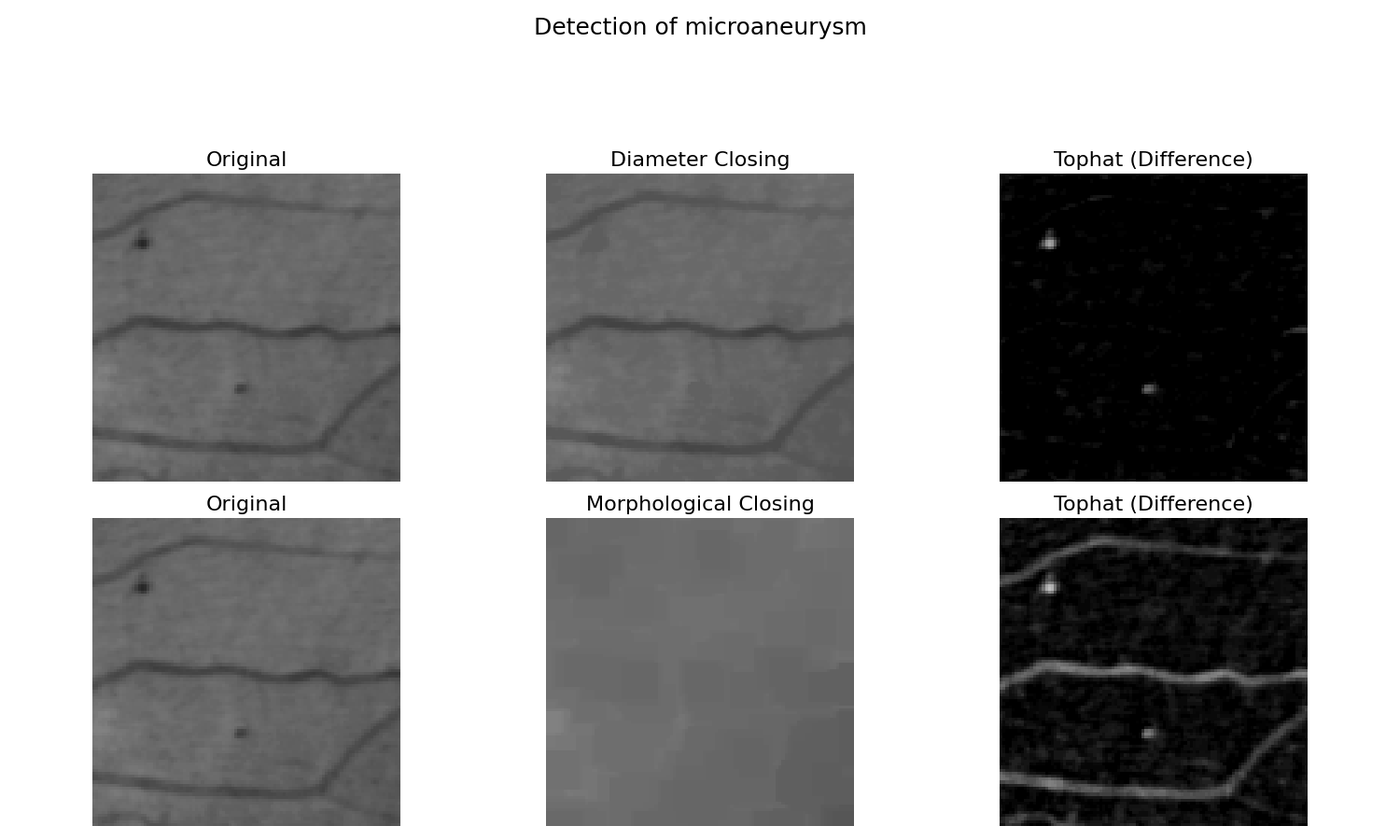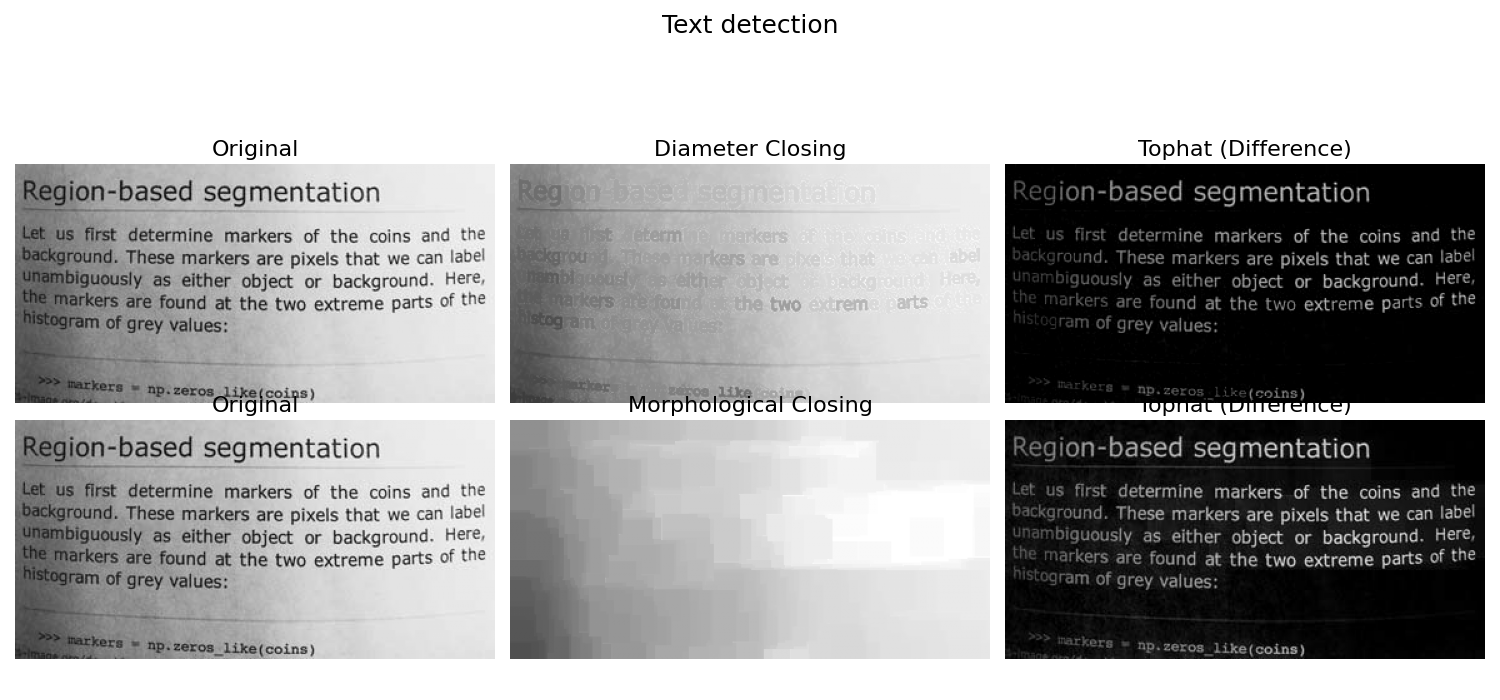# Attribute operators#

Attribute operators (or connected operators)  is a family of contour preserving filtering operations in mathematical morphology. They can be implemented by max-trees , a compact hierarchical representation of the image.

Here, we show how to use diameter closing  , which is compared to morphological closing. Comparing the two results, we observe that the difference between image and morphological closing also extracts the long line. A thin but long line cannot contain the structuring element. The diameter closing stops the filling as soon as a maximal extension is reached. The line is therefore not filled and therefore not extracted by the difference.

```import matplotlib.pyplot as plt
from skimage.morphology import diameter_closing
from skimage import data
from skimage.morphology import closing
from skimage.morphology import square

datasets = {
'retina': {'image': data.microaneurysms(),
'figsize': (15, 9),
'diameter': 10,
'vis_factor': 3,
'title': 'Detection of microaneurysm'},
'page': {'image': data.page(),
'figsize': (15, 7),
'diameter': 23,
'vis_factor': 1,
'title': 'Text detection'}
}

for dataset in datasets.values():
# image with printed letters
image = dataset['image']
figsize = dataset['figsize']
diameter = dataset['diameter']

fig, ax = plt.subplots(2, 3, figsize=figsize)
# Original image
ax[0, 0].imshow(image, cmap='gray', aspect='equal',
vmin=0, vmax=255)
ax[0, 0].set_title('Original', fontsize=16)
ax[0, 0].axis('off')

ax[1, 0].imshow(image, cmap='gray', aspect='equal',
vmin=0, vmax=255)
ax[1, 0].set_title('Original', fontsize=16)
ax[1, 0].axis('off')

# Diameter closing : we remove all dark structures with a maximal
# extension of less than <diameter> (12 or 23). I.e. in closed_attr, all
# local minima have at least a maximal extension of <diameter>.
closed_attr = diameter_closing(image, diameter, connectivity=2)

# We then calculate the difference to the original image.
tophat_attr = closed_attr - image

ax[0, 1].imshow(closed_attr, cmap='gray', aspect='equal',
vmin=0, vmax=255)
ax[0, 1].set_title('Diameter Closing', fontsize=16)
ax[0, 1].axis('off')

ax[0, 2].imshow(dataset['vis_factor'] * tophat_attr, cmap='gray',
aspect='equal', vmin=0, vmax=255)
ax[0, 2].set_title('Tophat (Difference)', fontsize=16)
ax[0, 2].axis('off')

# A morphological closing removes all dark structures that cannot
# contain a structuring element of a certain size.
closed = closing(image, square(diameter))

# Again we calculate the difference to the original image.
tophat = closed - image

ax[1, 1].imshow(closed, cmap='gray', aspect='equal',
vmin=0, vmax=255)
ax[1, 1].set_title('Morphological Closing', fontsize=16)
ax[1, 1].axis('off')

ax[1, 2].imshow(dataset['vis_factor'] * tophat, cmap='gray',
aspect='equal', vmin=0, vmax=255)
ax[1, 2].set_title('Tophat (Difference)', fontsize=16)
ax[1, 2].axis('off')
fig.suptitle(dataset['title'], fontsize=18)
fig.tight_layout(rect=(0, 0, 1, 0.88))

plt.show()
```
••